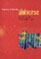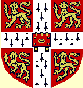© CAMBRIDGE UNIVERSITY PRESS 1999

3.3. The Intracluster Gas: Some Relevant Questions

Some of the fundamental questions about the intracluster gas relate to its origin, evolution, metal enrichment, hydrodynamical state in the cluster, and its relation to the distribution of galaxies and mass in the cluster. I list below some of the relevant questions and discuss a selection of these topics in the following subsections. Some of the questions posed do not yet have sufficient observational constraints to suggest a possible solution.

• What is the hydrodynamical state of the hot gas in clusters? Is it in approximate hydrostatic equilibrium with the cluster potential?

• What is the relation of the intracluster gas to the galaxies and mass in the cluster? For example (the subscripts below refer to gas, galaxies, and mass, respectively):

-- Density profiles:gas(r) vs.gal(r) vs.m(r)?

-- Temperature-velocity relation: T vs.gal vs.m?

-- Mass: Mgas vs. Mgal vs. Mcl?

-- Profiles of the above properties:
T(r) vs.gal(r) vs.m(r)?
Mgas(r) vs. Mgal(r) vs. Mcl(r)?

-- Core radii: Rc(gas) vs. Rc(gal) vs. Rc(m)?

-- Luminosity versus galaxy-type relation: Lx vs. spiral fraction?

• What is the origin of the hot intracluster gas?

• What is the origin of the metal enrichment of the gas?

• What is the evolution of the intracluster gas?